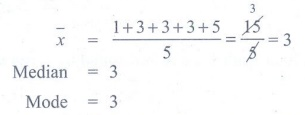Home | | Maths 9th std | Exercise 8.4: Multiple choice questions

# Exercise 8.4: Multiple choice questions

Maths : Statistics: Book Back, Exercise, Example Numerical Question with Answers, Solution: Exercise 8.4: Multiple choice questions, One Mark Choose the correct Answers

Exercise 8.4

Multiple choice questions

1. Let m be the mid point and b be the upper limit of a class in a continuous frequency distribution. The lower limit of the class is

(1) 2m − b

(2) 2m+b

(3) m-b

(4) m-2b.

2. The mean of a set of seven numbers is 81. If one of the numbers is discarded , the mean of the remaining numbers is 78 . The value of discarded number is

(1) 101

(2) 100

(3) 99

(4) 98.

Solution:3. A particular observation which occurs maximum number of times in a given data is called its

(1) Frequency

(2) range

(3) mode

(4) Median.

4. For which set of numbers do the mean, median and mode all have the same values?

(1) 2,2,2,4

(2) 1,3,3,3,5

(3) 1,1,2,5,6

(4) 1,1,2,1,5.

Solution:5. The algebraic sum of the deviations of a set of n values from their mean is

(1) 0

(2) n-1

(3) n

(4) n+1.

6. The mean of a,b,c,d and e is 28 . If the mean of ac and e is 24 , then mean of b and is_

(1) 24

(2) 36

(3) 26

(4) 34

Solution:

a+b+c+d+/ 5 = 28

a+c+e / 3 = 24

b+d / 2 = ?

a+b+c+d+= 5 × 28 = 140

a+c+e  = 3 × 24 = 72

b+d  = 68

b+d / 2 = 68/2 = 34

7. If the mean of five observations xx+2 , x+4 , x+6 , x+8 , is 11 , then the mean of first three observations is

(1) 9

(2) 11

(3) 13

(4) 15.

Solution:

[x + (x+2) + ( x+4 ) + ( x+6) + (x+8)] / 5 = 11

[5x+20] / 5 =11

5x+20 = 55

5x = 35

x=7

Mean of [x + (x+2) + ( x+4 ) ] / 3

(7+9+11) / 3 = 27/3 = 9

8. The mean of 5, 9, x, 17, and 21 is 13, then find the value of x

(1) 9

(2) 13

(3) 17

(4) 21

Solution:

[5+9+x+17+21] / 5 = [52+x]/5 = 13

52+x = 65

x = 65-52 = 13

9. The mean of the square of first 11 natural numbers is

(1) 26

(2) 46

(3) 48

(4) 52.

Solution:10. The mean of a set of numbers is. If each number is multiplied by z, the mean is

(1)+z

(2)− z

(3) z(4)Exercise 8.4

1. (1)  2. (3)  3. (3)  4. (2) 5. (1)  6. (4)  7. (1) 8. (2)  9. (2) 10. (3)

Project

1. Prepare a frequency table of the top speeds of 20 different land animals. Find mean, median and mode. Justify your answer.

2. From the record of students particulars of the class,

(i) Find the mean age of the class ( using class interval)

(ii) Calculate the mean height of the class( using class intervals)

Tags : Numerical Problems with Answers, Solution | Statistics | Maths , 9th Maths : UNIT 8 : Statistics
Study Material, Lecturing Notes, Assignment, Reference, Wiki description explanation, brief detail
9th Maths : UNIT 8 : Statistics : Exercise 8.4: Multiple choice questions | Numerical Problems with Answers, Solution | Statistics | Maths